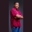Related Tags

python
prime
communitycreator

# How to find numbers that are prime in nth numbers in PythonTheodore Kelechukwu Onyejiaku

### What is a prime number?

A prime number is a number that is divisible only by itself and one. Prime numbers are different from odd and even numbers.

### How to find prime numbers in nth numbers

We can find the prime numbers present in a specified range of numbers, say 0-10, 0-50, and so on in Python by doing the following.

#### Step 1

Create the limit or nth number variable.

limit = 50


#### Step 2

Create a for loop that will loop for the limit specified. The range() method will be used.

for i in range(0, limit ):


#### Step 3

An if statement will be placed to check if the ith loop is greater than one. Of course, prime numbers do not begin with one.

if i > 1:


#### Step 4

Once the condition is true, another loop will run for a number of times equal to the ith value.

for j in range(2, i):


#### Step 5

And in the loop, we will use the modulus operator to check if the ith value when divided by the jth value will give a remainder of 0. If it is true then the loop terminates. Otherwise it prints out the prime number.

for j in range(2, i):
if (i % j) == 0:
break
else:
print(i)


### Code

Below is the complete code.

limit = 50

for i in range(0, limit):
# all prime numbers are greater than 1
if i > 1:
for j in range(2, i):
if (i % j) == 0:
break
else:
print(i)

RELATED TAGS

python
prime
communitycreator

CONTRIBUTORTheodore Kelechukwu Onyejiaku
RELATED COURSES

View all Courses

Keep Exploring

Learn in-demand tech skills in half the time